Uncertainty '96 (ASCE), University of Wisconsin, Madison, Wisconsin, August 1-3, 1996.

Evaluating Subsurface Uncertainty Using Zonal Kriging

Abstract

Kriging and Conditional Indicator Simulation are valuable tools for evaluating uncertainty in the subsurface, but are limited by the assumption of stationarity (the mean and variance directionally across the site are constant). Using a technique called Zonal Kriging, different spatial equations can be applied to separate regions of a site. If the regions are distinct and unrelated, then zonal kriging can be accomplished manually by modeling each region and merging the results into a single model. However, merging results is difficult and expensive in human resources and computer processing time, and merged results cannot represent gradational regions. A zonal kriging tool is presented and applied to both a synthetic data set and to data from an extensively sampled outcrop in Yorkshire, England. Estimations of the hypothetical data set demonstrate the advantages and shortcomings of the technique. Simulation of the Yorkshire outcrop using both zoned-indicator simulations and traditional simulations illustrate the improvement attained through use of zonal kriging.

Introduction

Significant variation of spatial statistics across a site, or non-random sampling can violate the basic assumptions of stationarity (Journel and Huijbregts, 1978) and this can lead to strongly biased estimates (Dagdelen and Turner, 1996). Depending on the magnitude of the variation and the importance of the results, two approaches are often taken. One assumes the problem can be controlled with the local stationarity of the neighboring data samples (Isaaks and Srivastava, 1989), and a spatial model which reflects some mean behavior of the entire site can be found. The other divides the area into an appropriate number of zones, describes the spatial statistics for each zone, estimates each zone, and merges the results. One problem with the second method is that the boundary between zones can be rough or abrupt (Kushnir and Yarus, 1992). This may be appropriate where the contact is a fault or an unconformity, but where the underlying geologic process yields transitional material, the results are unsatisfactory.

An alternative approach has been to transform all the points in the data set to match the spatial statistics of the cell currently being estimated (Kushnir and Yarus, 1992). Here all the site data are considered, whether they are from the zone of interest or not. This method eliminates the problem of rough zone boundaries, and addresses the possible gradation of properties between zones. The approach also eliminates the need to manually merge individual zones into a single model. However, it does not allow for sharp boundaries, nor does it recognize that some points from neighboring zones may have no bearing on the estimated value, even though they may be in the search neighborhood.

The approach described here merges the two zoning techniques described above and adds utilities to define inter-zone relationships. Such relationships describe how data, located in one zone are treated when sampled for a cell calculation located in another zone. This technique is applied using Simple (SK) and Ordinary Kriging (OK) (Journel and Huijbregts, 1978), and Conditional Indicator Simulation (CIS) (G—mez-Hern‡ndez and Srivastava, 1990). CIS is used in this paper in two ways. It is first used to define the boundaries of each zone. CIS can generate multiple, unique, realizations of the zone boundaries, which honor the statistics of the data. This makes the simulation a two step process; defining the location of zones with CIS, then estimating the values within each zone using SK or OK. The second use of CIS, is populating the zones with realistic, probabilistic, alternatives (individual realizations).

Methodology

To implement zonal kriging, existing kriging algorithms were modified (ktb3dm, Deutsch and Journel, 1992; and ISIM3D, G—mez-Hern‡ndez and Srivastava, 1990, and McKenna, 1994). Both codes have the required mathematical tools, but the calculation sequence was reordered, additional input was defined, and the search algorithm was modified. A flow chart for the standard kriging algorithm and the modifications for zonal kriging (italicized steps) are shown in the flow chart in Figure 1.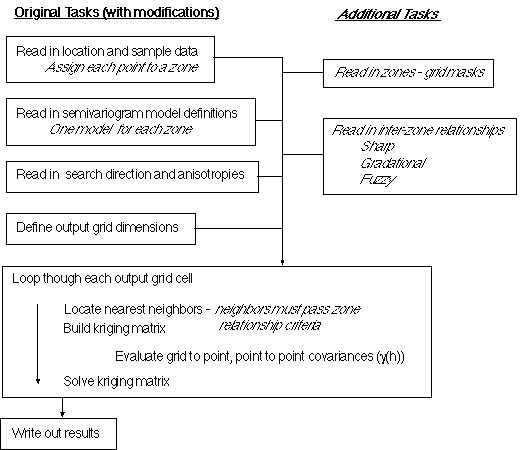Figure 1: Basic steps involved in standard kriging algorithm, with the additional steps needed to implement Zonal Kriging in italics.

The key new aspects are:

1) Define the zones: This may be the most arbitrary portion of the process. Choosing the location of boundaries between zones is subjective, particularly when the data are sparse. One of the examples described below, uses a realization from a Conditional Indicator Simulation (CIS) to define the zonation. By repeating the process using zones from a series of realizations, much of the uncertainty associated with the location of the boundary can be addressed (although this is not done in this paper). In the second example CIS is not used to define zones because there is adequate borehole control, and the zones are based on stratigraphic interpretation.

2) Define inter-zone relationships. These may be Sharp (the zones are completely unrelated), Gradational (one zone merges infinitely into the other), or Fuzzy (the zones are gradational over a limited distance and then the units are distinct). If they are Fuzzy (this term does not refer to fuzzy logic), the width of the boundary must also be defined. The rationale behind each is as follows:

Sharp: In many cases, two units are in contact with one another, but otherwise are unrelated. Examples are faults and geologic unconformities. In this situation, there is no reason to use data from one zone to estimate the spatial distribution in the other.

Gradational: In some environments, units grade into one another. This is typical of coastal deposits where beach sands grade into marine clays and shales. In each environment, the depositional systems are very different, but the change is gradational. This method assumes that the region being estimated lies fully within the gradational region.

Fuzzy: This option is similar to the Gradational method. The difference is that the transitional distance is limited in extent. Beyond a defined distance, data from the other zone is no longer correlated to the location being considered. The width of this boundary is subjective, as it is not necessarily related to the range of the semivariogram for either zone. Defining the width of the zone is left to the modeler, and is based on their experience and knowledge of site conditions. In general the range of the shortest semivariogram may be reasonable. Note, the Gradational method, is the same as the Fuzzy method with an infinite boundary width.
Once 1) and 2) are defined, the algorithm:
3) Assigns each data point to a zone.

4) Creates a mask for each zone, describing how each cell within the grid will be treated.
Once these prerequisite data are defined, then each grid cell can be evaluated. When estimating a grid cell value, the modified programs use the properties associated with the zone in which that cell lies. This includes search criteria and semivariogram model information. The algorithm then:
5) Finds the nearest neighboring data points that fall 1) within the zone being evaluated, 2) within another zone which is gradational to the zone being evaluated, and 3) within the fuzzy boundary of the neighboring zone. All other points are ignored.

6) Generates and solves the kriging matrix. Once the nearest neighbor points have been selected, the kriging algorithm proceeds, evaluating all points using the semivariogram model from the zone of the cell being estimated. This is regardless of what zone each point is from, or how deep the point is into the neighboring zone. The information describing the semivariogram from the neighboring zone is ignored because merging the models can lead to a matrix that is not positive definite, a basic assumption for kriging (Myers and Journel, 1990).

Examples

Two examples are used to demonstrate the utility of zonal kriging. The first is based on a small sample of eleven synthetic data points. This example demonstrates 1) the differences between Simple Kriging (SK) and SK with zoning; 2) how different inter-zone relationships can effect results; and 3) some of the shortcomings of zonal kriging. The second example applies zonal kriging and indictor simulation to an extensively sampled fluvio-deltaic outcrop in Yorkshire, England (Ravenne et al, 1987). This outcrop exhibits two zones with different spatial characteristics, and was "sampled" on seven vertical lines representing bore-holes. This allowed the comparison of simulations based on a small sample of points, to the full, known section. This example demonstrated that dividing the cross-section into two zones yielded more realistic realizations as compared with modeling the site using a single zone.

Synthetic Data Set Example

A simple, synthetic, two-dimensional data set of elevations with eleven data points (Figure 2a) was evaluated using SK (Figure 2b). If the spatial statistics of the site are relatively consistent, this may be a good interpretation. However, if the data reflect material properties from three distinctly different units, where the spatial statistics are substantially different, another interpretation is needed (three zones is excessive given the amount of data, but is useful for this demonstration). Given a map of the material zones (Figure 2c is one possible zonation realization created using CIS) the site can be modeled using one of several assumptions: 1) the zones are completely unrelated; 2) there is an infinite gradation between zones; or 3) there is a short distance over which the zones are gradational. For these examples, the following semivariogram models were used:

Single Zone Zone 1 Zone 2 Zone 3
Model Type Spherical Spherical Spherical Spherical
Range 150 m 150 m 175 m 200 m
C1 0.24 0.24 0.22 0.22
Nugget 0.01 0.01 0.03 0.03

All models are isotropic. Although these models are not dramatically different, some interesting results are obtained.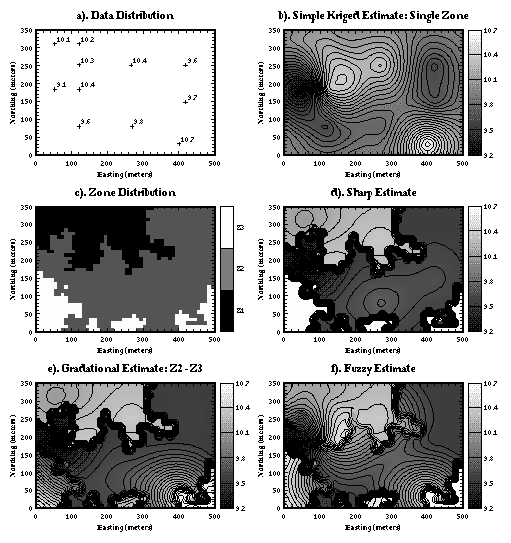Figure 2:Different forms of ordinary and zonal kriging. (a) Sample data, (b) a traditional Simple Kriged map, (c) one possible zone map from a conditional indicator simulation, (d) sharp transition, (e) gradational transition, (f) fuzzy transition.

Simple kriging with sharp, non-gradational contacts yields the map shown in Figure 2d. In this model, Zone 1 and 3 were gradational, but Zone 2 was completely unrelated. This yields sharp transitions between Zone 2 and the other two zones. Within each zone though, the surfaces are smooth as would be expected with SK. Note that the sharp transition matches the zone definition (Figure 2c).

A gradational contact between adjacent units produces a different map. In this example (Figure 2e), Zones 1 and 3 are still gradational, and the contact between Zones 2 and 3 is defined as gradational too (the contact between Zone 1 and 2 is sharp). The contour lines in Zone 1 are unchanged (Figure 2d), but there is substantial "smoothing" between Zones 2 and 3, although it is not complete, and the boundaries are somewhat abrupt. This incomplete smoothing occurs because the data control for neighboring cells in different zones are coupled with different semivariogram models, and because the model range is very near the sample spacing of the data. These rough transitions will disappear with finer sampling.

The final option is explored by defining transitional (fuzzy) contacts of finite thickness. In this example, Zone 1 was defined to have a fuzzy boundary of 20 meters with Zone 2, and Zone 3 was defined to have a fuzzy boundary of 40 meters with Zone 2. Although there is substantial smoothing, each zone maintains much of its own character (Figure 2f), and the map is still substantially different than that generated using single zone SK (Figure 2b).

Which of the above models actually best represents the synthetic site is not an issue for this presentation. What is important, is the modeler can control zonal differences and inter-relationships as appropriate for the site under evaluation, providing additional flexibility. Neither SK, nor OK could generate anything other than the first map (Figure 2b) or the second map if results were merged manually (Figure 2d).

As shown in Figures 2e and 2f, gradational and fuzzy boundaries can be abrupt. This is a numerical feature and not physical feature. The problem occurs when the sample data control between neighboring grid cells is substantially and consistently different. In this model, this occurs because sample spacings are close to the range of the zonal semivariogram models. If the site was modeled with 1) more data points (evenly scattered), or 2) with longer semivariogram model ranges, the contacts would be less abrupt.

Yorkshire, England Example

An outcrop cross-section from a fluvio-deltaic deposit near Yorkshire, England was sampled on a 17 x 20 cm spacing (Ravenne et al, 1987). For practical reasons (computation time) we upscaled the data to 2m x 1m grid blocks (McKenna and Poeter, 1994). The full 600m x 30m cross-section is shown in Figure 3a, and is composed of three materials: shale (SH), shaley-sandstone (SH-SS), and sandstone (SS).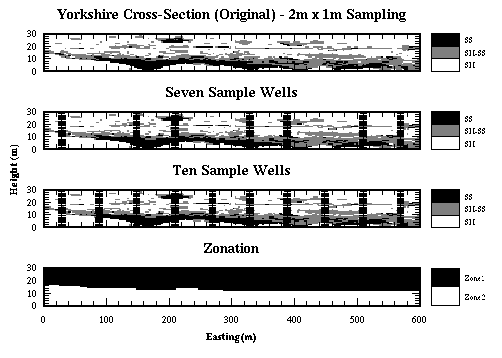Figure 3: Model definition information for the Yorkshire cross-section: a) actual cross-section sampled with 2m x 1m cells; b) and c) the locations of 7 and 10 "well samples;" d) zone definition.

Simple kriging with sharp, non-gradational contacts yields the map shown in

To demonstrate the advantages of zonal kriging, the cross-section was "sampled" on seven vertical lines representing wells (Figure 3b) (1m vertical samples). Two distinct zones exist: a lower zone with high continuity in the SS and SH-SS units, and an upper zone dominated by SH, with small lenses of SS and SH- SS. To confirm that the spatial statistics indeed were different, the original section was divided into two zones (Figure 3d) based of a fence diagram of the bore-hole data. The facies frequencies and semivariograms for the entire cross-section were compared with those from the two zones. Whether examining the data from the exhaustive data set of the full cross-section or the well samples, the results are similar; about 61% of the samples are SH, 24% SH-SS, and 15% SS. When the individual zones are separated, the distributions are very different:

SH (%) SH-SS (%) SS (%)
Cross-Section (All) 63.4 23.6 13.0
Wells (All) 59.0 24.8 16.2
Cross-Section (Top) 81.2 16.4 2.4
Cross-Section (Bottom) 40.2 32.9 26.9
Wells (Top) 80.7 15.8 3.5
Wells (Bottom) 37.2 32.6 30.2

The top zone is contains more than twice as much SH as the bottom zone and almost no SS, whereas the materials are more evenly distributed in the bottom zone. When semivariograms are calculated for the entire section, and for the top and bottom zones, using both the full cross-section and the well data, the zonation is again apparent. The horizontal and vertical indicator semivariograms are shown in Figures 4 through 7. The spatial statistics of the top zone are clearly different than those of the bottom zone, and those of the entire cross-section. The horizontal range for the SH/SH-SS threshold in the top zone is only about 15% to 25% that of the bottom zone (Figures 4 and 6). For the SH-SS/SS threshold, the horizontal range in zone 1 is about 35% of that in zone 2 (Figures 5 and 7).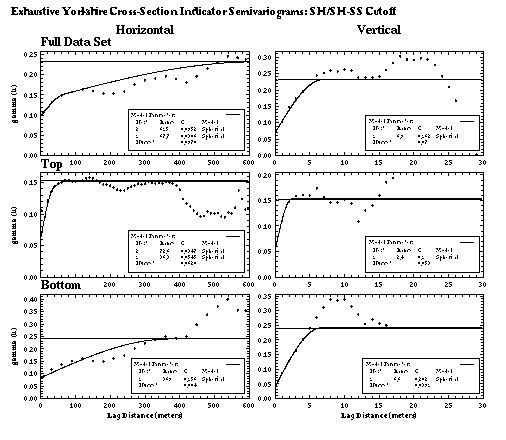Figure 4: Experimental and model indicator semivariograms for SH/SH-SS cutoff (full cross-section).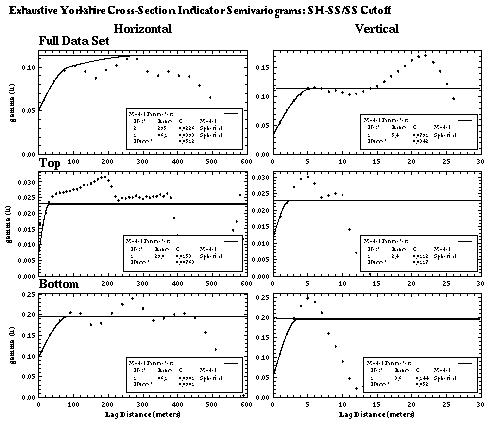Figure 5: Experimental and Model indicator semivariograms for SH-SS/SS cutoff (full cross-section).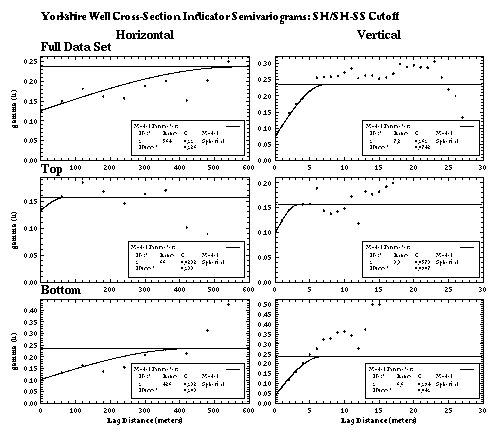Figure 6: Experimental and Model indicator semivariograms for SH/SH-SS cutoff (well data).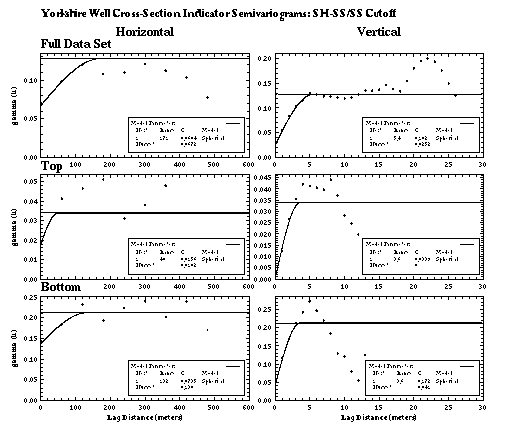Figure 7: Experimental and Model indicator semivariograms for SH-SS/SS cutoff (well data).

The assumption of stationarity is not applicable to this cross-section, thus modeling this site using a single set of semivariograms is statistically inappropriate. Two ensembles of 100 realizations each, demonstrate that zonal kriging yields more accurate results than a single semivariogram model. The first ensemble is based on the assumption that stationarity is valid, and only one semivariogram model set is required (the full well data set semivariogram models, Figures 6 and 7). The second set is based on the observation that stationarity is violated between zones, but is valid within each zone, thus a sharp transition is assumed, and the "Top" and "Bottom" semivariogram models (Figures 6 and 7) are used. The ranges of both horizontal and vertical semivariograms, in the top zone are much shorter than the ranges for the full section and the bottom zone. The model grid matches the original cross-section grid exactly; with cells 2m x 1m in 300 columns and 30 layers. Results of several realizations using one and two zones respectively, are shown in Figures 8 and 9. Differences between the two sets are subtle, but discernible in the top zone. There are differences in the bottom zone, but they are minor. To evaluate the results, realizations were compared in order (Realization #1 [Zone 1] vs. #1 [2 Zones], #2 [Zone 1] vs. #2 [2 Zones], etc.) because matched realizations use the same "random" search path to evaluate the grid, and start using the same random seed. Therefore differences are only attributed to the use of zoning, and the realization with the lowest mean-square error (MSE), is the more accurate model. The MSE was calculated by subtracting the actual grid value from the estimated grid value at each grid location, squaring that residual, summing the squared residuals for all cells and dividing by the number of model realizations.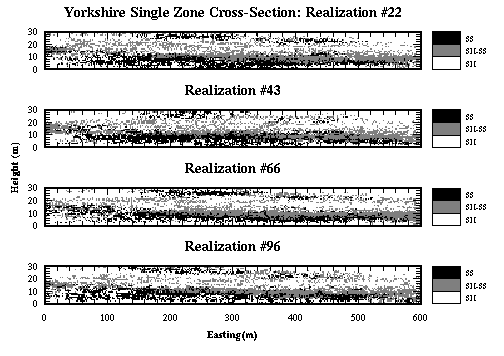Figure 8: Results from single zone simulation series.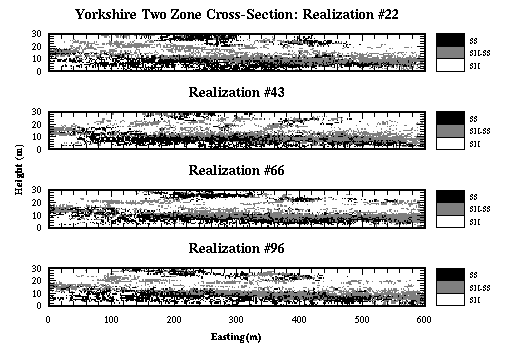Figure 9: Results from two zone simulation series.

In the four realizations shown (using either method), there is a largely continuous SH-SS/SS unit in the bottom zone, which is present in the actual section, though there are random fluctuations in the realizations. The random fluctuations in the realizations are due to the large nugget terms in the model semivariograms. The difference between the lower portions of the two series of simulations is minor, because the semivariogram models are fairly similar for this portion of the model.

There are greater differences between the one and two zone simulations in the top zone. Both exhibit a great deal of randomness due to large nuggets, but the results using two zones create more solid lenses without as many small isolated cells (Compare Realizations #66, this was one of the most accurate models, and #22 one of the least accurate). The single zone model tends to create long, thin layers with considerable scatter.

One-hundred pairs of realizations (one zone and two zone) were generated using the same random number sequence, and random path through the model grid. These pairs were compared to the actual cross-section. Realizations generated with the two zone approach had lower MSEŐs in 74 of the 100 pairs. When ten equally spaced wells (Figure 3c) were used instead of the seven shown (Figure 3b), realizations generated with the two zone approach had lower MSEŐs in 91 of the 100 pairs. The improved MSEŐs indicate that modeling the site with two zones yields better results. Although more work is involved in setting up the model (additional semivariogram calculations, data entry, zone definition), results are improved.

In addition to improved accuracy, the modeler has more flexibility in fine tuning the model solutions for each zone. The results can be dramatic in one zone without affecting the other. A sample two-zone realization is shown in Figure 10a. Realizations resulting from independently decreasing the nuggets in the top and bottom zones are shown in Figures 10b and 10c respectively. In both cases, the continuity is increased and the random scatter is reduced, without affecting the alternate zone. If these same changes were made on a single zoned realization, they would effect the entire grid, and improvement in one portion of the grid would have to be balanced against the degradation of the other zone.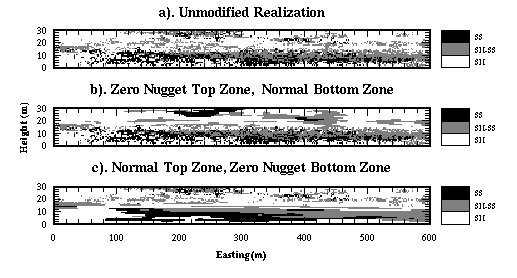Figure 10: Impact of altering the semivariogram nugget independently in the top and bottom zones of a section: a) original simulation section; b) reduced nugget in top zone; c) reduced nugget in bottom zone.

Conclusions

Through a series of examples, we have shown that zonal kriging can yield significantly different results than are obtained using SK or conditional indicator simulation alone. At sites where the assumption of stationarity is invalid, when correctly applied, zonal kriging will produce realizations that more accurately represent reality. The technique requires additional data processing to define the model, and unusual boundary effects may occur when sample data are sparse or are located at spacings near the range of the semivariogram models. These shortcomings are balanced by the improved accuracy and flexibility in modeling.

References

Dagdelen, K, and A.K. Turner, 1996, Importance of Stationarity for Geostatistical Assessment of Environmental Contamination, Geostatistics for Environmental and Geotechnical Applications, ASTM STP 1283, R.M. Srivastava, S. Rouhani, M.V. Cromer, A.I. Johnson, Ed., American Society For Testing and Materials, Philadelphia.

Deutsch, C.V., and A.G. Journel, 1992, GSLIB: Geostatistical Software Library and User's Guide, Oxford University Press, New York.

Gomez-Hernandez, J.J. and R.M. Srivastava, 1990, ISIM3D: An ANSI-C Three Dimensional Multiple Indicator Conditional Simulation Program, Computers in Geoscience, Vol. 16, No. 4, pp. 395-440.

Isaaks, E.H., and R.M. Srivastava, 1989, An Introduction to Applied Geostatistics, Oxford University Press, New York.

Journel, A.G. and Huijbregts, 1978, Mining Geostatistics, Academic Press, London.

Kushnir, G., J.M. Yarus, 1992, Computer Modeling of Geologic Surfaces and Volumes, AAPG Computer Applications in Geology 1, Modeling Anisotropy in Computer Mapping of Geologic Data, The American Association of Petroleum Geologists, Tulsa, pp 75-92.

McKenna, S.A, and E.P. Poeter, 1994, Simulating Geological Uncertainty with Imprecise Data for groundwater Flow and Advective Transport Modeling, Stochastic Modeling and Geostatistics: Principles, Methods, and Case Studies, Edited by J.M. Yarus and R.L. Chambers: AAPG Computer Applications in Geology, Association of Petroleum Geologists, Tulsa, pp 241-248.

Myers, D.E., and A.G. Journel, 1990, Variograms and Zonal Aniostropies and Noninvertable Kriging Systems, Mathematical Geology, Vol. 22, No. 7, pp 779- 785.

Ravenne, C., R. Eschard, A. Galli, Y. Mathieu, L. Montadert, and J.L. Rudkiewicz, 1987, Heterogeneities and Geometry of Sedimentary Bodies in a Fluvio-Deltaic Reservoir, SPE 16753, SPE Annual Technical Conference and Exhibition, Dallas TX, pp 115-124.